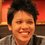# Find the simplest way to show that there exist positive integers $x,y,z$ that satisfy the equation $29x +30y+31z=366366$.

.Note by Pi Han Goh
6 years, 3 months ago

This discussion board is a place to discuss our Daily Challenges and the math and science related to those challenges. Explanations are more than just a solution — they should explain the steps and thinking strategies that you used to obtain the solution. Comments should further the discussion of math and science.

When posting on Brilliant:

• Use the emojis to react to an explanation, whether you're congratulating a job well done , or just really confused .
• Ask specific questions about the challenge or the steps in somebody's explanation. Well-posed questions can add a lot to the discussion, but posting "I don't understand!" doesn't help anyone.
• Try to contribute something new to the discussion, whether it is an extension, generalization or other idea related to the challenge.

MarkdownAppears as
*italics* or _italics_ italics
**bold** or __bold__ bold
- bulleted- list
• bulleted
• list
1. numbered2. list
1. numbered
2. list
Note: you must add a full line of space before and after lists for them to show up correctly
paragraph 1paragraph 2

paragraph 1

paragraph 2

[example link](https://brilliant.org)example link
> This is a quote
This is a quote
    # I indented these lines
# 4 spaces, and now they show
# up as a code block.

print "hello world"
# I indented these lines
# 4 spaces, and now they show
# up as a code block.

print "hello world"
MathAppears as
Remember to wrap math in $$ ... $$ or $ ... $ to ensure proper formatting.
2 \times 3 $2 \times 3$
2^{34} $2^{34}$
a_{i-1} $a_{i-1}$
\frac{2}{3} $\frac{2}{3}$
\sqrt{2} $\sqrt{2}$
\sum_{i=1}^3 $\sum_{i=1}^3$
\sin \theta $\sin \theta$
\boxed{123} $\boxed{123}$

Sort by:

Number of months with $29 , 30$ and $31$ days in $1001$ leap years.

- 6 years, 3 months ago

Wow that's quick!

- 6 years, 3 months ago

Thanks.

- 6 years, 3 months ago

awesome...

- 6 years, 3 months ago

Genius!

- 6 years, 3 months ago

Cool observation Sir!

- 6 years, 3 months ago

amazing !

- 6 years, 3 months ago

Take $x=1$ and by Chicken McNugget there exists $y,z$ such that $30y+31z=366337$.

That's the most straightforward way that immediately solves the problem as far as I know.

- 6 years, 3 months ago

But Chicken McNugget didn't explicitly say that $y,z$ are positive.

- 6 years, 3 months ago

It does; or else it would just degenerate to Bezout's Identity.

- 6 years, 3 months ago

OH wait it does! Silly me! Thanks! I've found the second simplest solution. Yay!

- 6 years, 3 months ago

x=3955;y=4078;z=4171

- 6 years, 2 months ago

x=3955;y=4078;z=4171;

- 6 years, 2 months ago

see if HCF of 29,30,31 divides 366366 completely

- 6 years, 3 months ago

Won't HCF of $29$, $30$ and $31$ be $1$?

- 6 years, 2 months ago